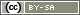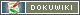# Joint Randomization of Different Stimuli

Sometimes experimetalgroups get more than one stimulus. For exmple the group A gets the text A and the image A while the group B gets a text B and an image B presented. This Chapter explains in an example how different stimuli can be randomized together per PHP code.

Note: If you want to randomize a question and a stimulus together, please read the chapter Randomization: Questions. This chaper explains already in detail how to randomize a question and a stimuli, for exaple an image, together.

## Example: Texts and Mediafiles together

The following PHP code should be known already (s. Randomization: Texts):

```if (value('RG01') == 2) {
text('ST01');
} elseif (value('RG01') == 3) {
text('ST02');
}```

This PHP code presents depending on the drawn note by the random generator “RG01” a text “ST01” or “ST02” and the controlgroup “RG01=01” gets no text.

Now, you may want to present the participants of a group not only one but more stimuli, depending on the assigned group.

### Several Stimuli on One Page

For this purpose, you can simply append another text or stimulus to the text() in the PHP code to the condition drawn in the random generator:

```if (value('RG01') == 2) {
text('ST01');
text('IM01');
} elseif (value('RG01') == 3) {
text('ST02');
text('IM02');
}```

In this example, the group with the drawn random number “RG01=2” would have the text “TX01” and the image “IM01” and the group with the random number “RG01=3” would have the text “TX02” and the image “IM01” displayed on one page. The control group “RG01=1” again gets no stimulus dispayed.

Note: In our example the texts() with the IDs “IM01” sad “IM02” are images but it could also be other media files (audios and videos): Media Files in Questionnaires (Audio, Video, Documents) and Randomization Mediafiles.

### Several Stimuli on Different Pages

If you want to display various stimuli to the groups on different pages, you can read the random number several times on different pages. To accomplish this, place the appropriate PHP codes to present the stimuli on the pages you want to use. However, the random number generator may only be placed once before the first PHP code during Compose Questionnaire, so the random number will not change.

Example:

```// Code on the page, where the first stimulus is presented
if (value('RG01') == 2) {
text('ST01');
} elseif (value('RG01') == 3) {
text('ST02');
}```
```// Code on the page, where the other stimulus is presented
if (value('RG01') == 2) {
text('IM01');
} elseif (value('RG01') == 3) {
text('IM02');
}```

The groups are again shown the same stimuli as above but the stimuli can now be placed on different pages in the questionnaire.